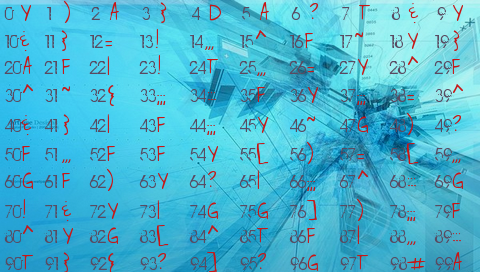Mind Reader by French developer walook is a bit hard to describe, so let’s quote the author:

Choose an integer number between 1 and 99!

Let’s take for example 67:

Subtract from this number each of the two digits that make it.
In our example, The result is: 67 – 6 – 7 = 54
Look at the table : Each symbol corresponds to a number.
Find the symbol corresponding to yours.
Finally, press cross!http://wololo.net/talk/viewtopic.php?f=26&t=6363